Dim

Creates horizontal or vertical linear dimensions.

Steps:

1. Pick

the first point.

2. Pick the second point.

3. Pick the dimension line location.

4. Note: Dimensions always measure as though the object were projected to the current construction plane.

Options

Style

Enter the dimension style
name.

Vertical

Draws the dimension aligned with the construction plane y-axis.

Horizontal

Draws the dimension aligned with the construction plane x-axis.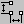Standard > Linear Dimension Dimension > Linear DimensionDimension > Linear Dimension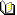Related topics…

DimAligned

Creates a linear dimension lined up with two points.

Steps:

1. Pick

the first point.

2. Pick the second point.

3. Pick the location.

Options

Style

Enter the dimension style
name.Dimension > Aligned DimensionDimension > Aligned DimensionRelated topics…

DimAngle

Dimensions the angle between two lines.

Steps:

1. Select

a line.

2. Select a second line.

3. Pick

the dimension location.

4. Note: You can dimension polyline segments and linear surface and polysurface edges.

Options

Style

Enter the dimension style
name.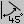Dimension > Angle DimensionDimension > Angle DimensionRelated topics…

DimDiameter

Dimensions the diameter of a curve.

Steps:

1. Pick a curve.

2. Pick the dimension location.

3. Note: Dimensions always measure as though the object were projected to the current construction plane.

Options

Style

Enter the dimension style
name.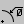Dimension > Diameter DimensionDimension > Diameter DimensionRelated topics…

DimOrdinate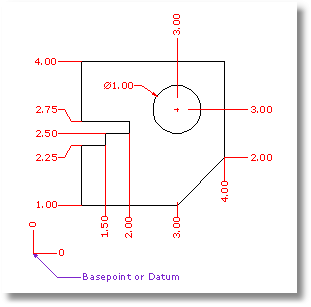Creates dimensions from an origin point to a feature.

Ordinate dimensions show the horizontal or vertical distance from an origin point (called the basepoint) to a dimensioned feature, such as a hole center or a feature in a part. Ordinate dimensions are widely used in CNC related manufacturing industries because the clutter caused by dimensions is minimized. This type of dimension prevents accumulated errors by showing the X or Y offset of the feature from the basepoint.

Steps:

1. Pick

a dimension point.

2. An XDatum dimension is implied if your second pick is primarily above or below the first pick.

A YDatum dimension is implied if your second pick is primarily left or right from the first pick.

4. Press Enter

when finished placing dimensions.

5. Note: The shape of the ordinate leader can be point edited after creation to avoid overlapping geometry.

Options

Style

Enter the dimension style
name.

XDatum

Overrides the implied biasing and forces an X-ordinate dimension.

YDatum

Overrides the implied biasing and forces a Y-ordinate dimension.

Basepoint

Changes the basepoint for the duration of the command. The basepoint reverts to the default construction plane origin when the DimOrdinate command is run again.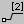Dimension > Ordinate dimensionDimension > Ordinate DimensionRelated topics…

Dimension a radius of an arc or circle.

Steps:

1. Select

a curve.

2. Pick

a dimension location.

Notes

• Dimensions always measure as though the object were projected to the current construction plane.

Options

Style

Enter the dimension style
name.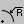Dimension > Radial DimensionDimension > Radial DimensionRelated topics…

DimRotated

Creates a linear dimension that is rotated from the x-y axis.

Steps:

1. Pick

two points to establish a rotation angle.

2. Pick the first dimension point.

3. Pick the second dimension point.

4. Pick the dimension location.

Options

Style

Enter the dimension style
name.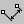Dimension > Rotated DimensionDimension > Rotated DimensionRelated topics…

DimRecenterText

Returns dimension text that has been moved back to its default location.

Steps:

• Select

dimensions.

• Note: To move dimension text away from the dimension line, turn on the dimension control points
and drag the text control point.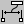Dimension > Recenter Dimension TextDimension > Recenter Dimension TextRelated topics…

Steps:

1. Pick

2. This is the arrow end.

3. Pick the next points of the leader.

4. Press Enter

to end the command.

5.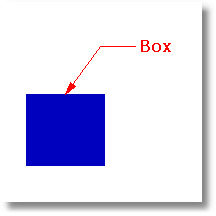Note: Dimension options
control the text height and arrow size.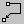Dimension > LeaderDimension > LeaderRelated topics…

Creates or removes an annotation arrowhead on the end of a curve.

Steps:

• Pick

a curve near the end where you want to place an arrowhead.

• Note: The arrowhead size cannot be changed.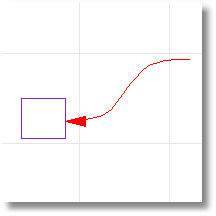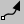Annotate > Add/Remove Arrowhead to CurveNoneRelated topics…